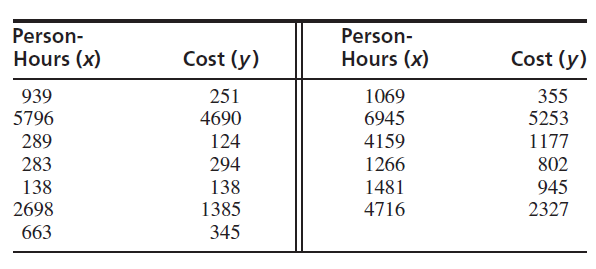×
Get Full Access to Statistics For Engineers And Scientists - 4 Edition - Chapter 7.4 - Problem 5e
Get Full Access to Statistics For Engineers And Scientists - 4 Edition - Chapter 7.4 - Problem 5e

×

# Good forecasting and control of preconstruction activitiesISBN: 9780073401331 38

## Solution for problem 5E Chapter 7.4

Statistics for Engineers and Scientists | 4th Edition

• Textbook Solutions
• 2901 Step-by-step solutions solved by professors and subject experts
• Get 24/7 help from StudySoup virtual teaching assistantsStatistics for Engineers and Scientists | 4th Edition

4 5 1 277 Reviews
28
1
Problem 5E

Good forecasting and control of preconstruction activities leads to more efficient use of time and resources in highway construction projects. Data on construction costs (in $1000s) and person-hours of labor required on several projects are presented in the following table and are taken from the article “Forecasting Engineering Manpower Requirements for Highway Preconstruction Activities” (K. Persad, J. O’Connor, and K.Varghese, Journal of Management Engineering, 1995:41–47). Each value represents an average of several projects, and two outliers have been deleted.a. Compute the least-squares line for predicting y from x. b. Plot the residuals versus the fitted values. Does the model seem appropriate? c. Compute the least-squares line for predicting ln y from ln x. d. Plot the residuals versus the fitted values. Does the model seem appropriate? e. Using the more appropriate model, construct a 95% prediction interval for the cost of a project that requires 1000 person-hours of labor. Step-by-Step Solution: Step 1 of 3 Chapter 9: Multivariate Designs - Means many variables - Study more than 2 variables in a single study - Enhance understanding of bivariate associations Longitudinal design - Measures same variables and same sample at several time points - Use to assess variable relationships across time - Use to see variable stability across time - 3 types of correlations assessed o Cross sectional: two variables measured at the same point in time [bivariate correlations] o Autocorrelations; testing variable against itself measured at two time points. o Cross-lag correlations; early measure of one variable against later measure of the other variable Moderation Language - Violence on TV vs. violence on TV with the parents - As longitudinal design; cross-sectional correlation o TV violence 3 grade & aggression 3 grade : r=.21 th th o TV violence 13 grade & aggression 13 grade : r=.05 - Auto correlational o TV violence 3 & TV violence 13 grade : r=.05 o Aggression 3 grade and aggression 13 grade : r=.35 - Cross-lag correlation rd th o TV violence 3rdrade & aggression 13 gradth: r=.31 o Aggression 3 grade & TV violence 13 grade : r=.01  Tells us that violence came first  Aggression doesn’t lead to them watch violence  Watch Step 2 of 3 ###### Chapter 7.4, Problem 5E is Solved Step 3 of 3 ##### Textbook: Statistics for Engineers and Scientists ##### Edition: 4 ##### Author: William Navidi ##### ISBN: 9780073401331 This full solution covers the following key subjects: preconstruction, model, appropriate, Projects, activities. This expansive textbook survival guide covers 153 chapters, and 2440 solutions. The full step-by-step solution to problem: 5E from chapter: 7.4 was answered by , our top Statistics solution expert on 06/28/17, 11:15AM. Statistics for Engineers and Scientists was written by and is associated to the ISBN: 9780073401331. This textbook survival guide was created for the textbook: Statistics for Engineers and Scientists , edition: 4. Since the solution to 5E from 7.4 chapter was answered, more than 367 students have viewed the full step-by-step answer. The answer to “?Good forecasting and control of preconstruction activities leads to more efficient use of time and resources in highway construction projects. Data on construction costs (in$1000s) and person-hours of labor required on several projects are presented in the following table and are taken from the article “Forecasting Engineering Manpower Requirements for Highway Preconstruction Activities” (K. Persad, J. O’Connor, and K.Varghese, Journal of Management Engineering, 1995:41–47). Each value represents an average of several projects, and two outliers have been deleted. a. Compute the least-squares line for predicting y from x.b. Plot the residuals versus the fitted values. Does the model seem appropriate?c. Compute the least-squares line for predicting ln y from ln x.d. Plot the residuals versus the fitted values. Does the model seem appropriate?e. Using the more appropriate model, construct a 95% prediction interval for the cost of a project that requires 1000 person-hours of labor.” is broken down into a number of easy to follow steps, and 146 words.

Unlock Textbook Solution